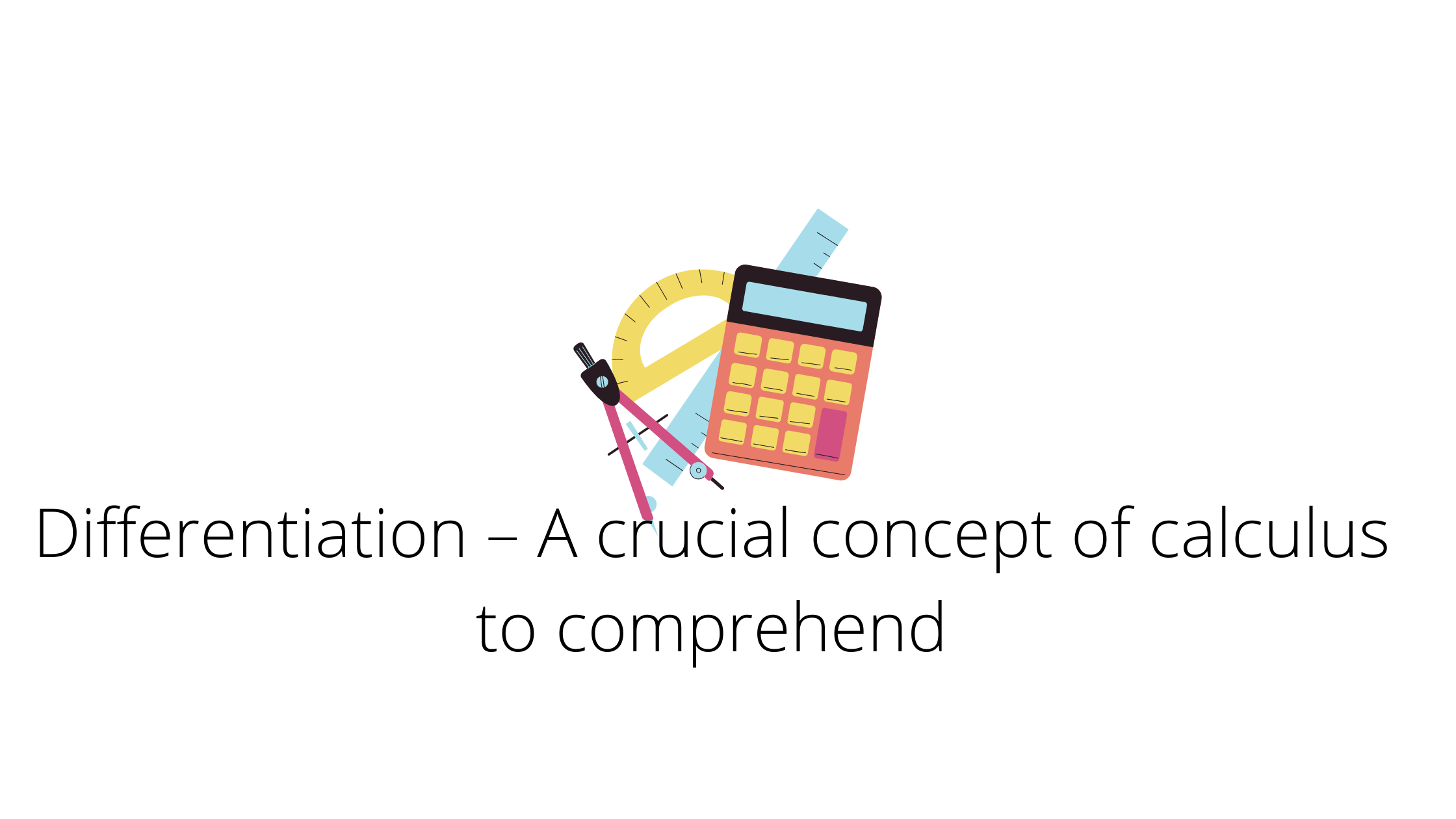# Differentiation – A crucial concept of calculus to comprehend

Calculus is a very significant and broad topic in mathematics. It has a wide range of real-world applications, making it crucial to grasp this subject. Calculus is a concept that most students are apprehensive about. Many of the concepts in calculus are difficult to grasp, one of them is differentiation. In this article, we’ll go over differentiation and a few of its formulas, such as the product rule.

## Differentiation

Differentiation is an important calculus idea. One important factor to remember is that the differentiation of a constant term is always zero. In general, differentiation refers to the pace at which one quantity changes with another. Differentiation has the amazing characteristic of allowing us to identify the expression’s maximum and minimum value. The slope of the tangent of the curve we’re differentiating is given by the geometric mean of differentiation. Assume that y is a parameter or function that is influenced by x. A little change in y is represented by dy, and a minor change in x is represented by dx. As a result, the differentiation formula dy/dx denotes that the difference in y is divided by the difference in x. Because d isn’t a variable, in this case, it can’t be wiped out. dy/dx is one of the most common ways of representing differentiation. Let us discuss a few of the formulas used in differentiation.

## Differentiation formula

Differentiating different quantities can be done in a variety of ways. If there is just one variable, it is differentiated with respect to that variable by subtracting one from the variable’s power and multiplying the original power by the quantity obtained after subtracting the power. Let’s say we’re given x4 to differentiate with. We’ll just reduce its power by one, yielding x3, and then multiply the original power of 4 by x3. As a result, the final result will be 4×3, which is the differentiation of x4.

Let’s have a look at how to differentiate an expression when it’s presented as the product of two or more variables. In these situations, we apply the product rule. Let’s say we have the formula f(x)*g(X) that needs to be differentiated in terms of x. To overcome this problem, we’ll apply the product rule. The product rule specifies that we must first differentiate any one expression, in this case, f(x), and then label the differentiated word as F. (x). Multiply F(x) by g(x), which is another variable term in (x). Now, in the provided example, we will differentiate the other term g(x) to get G(x) and multiply it by the first term, which is G(x)*f (x). Finally, combine the variables F(x)g(x) and G(x)f(x), resulting in F(x)g(x)+G(x)f(x). If more terms are given in the product form in the expression that has to be differentiated, we shall repeat the process.Another crucial formula of differentiation is the quotient rule.

Quotient rule: Whenever we are given a function in the form of a fraction and is to be differentiated, we use the quotient rule. In the quotient rule, we first differentiate the numerator and multiply it by the denominator. In the second step, we differentiate the denominator and multiply it with the numerator, and add both the quantities. And at last, we divide the sum by the square of the denominator. It is one of the most effective methods of dealing with functions that are in the form of a fraction.

These topics are crucial for students to understand because they are commonly used in higher education. If a student is having trouble solving a math-related problem, they should visit Cuemath. It is an online platform that provides high-quality education in math and coding to everyone in various countries.#### Sonal Shukla

View all posts by Sonal Shukla →